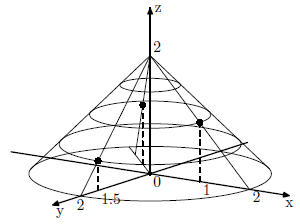## Problem D. Dome of Circus

A travelling circus faces a tough challenge in designing the dome for its performances. The circus has a number of shows that happen above the stage in the air under the dome. Various rigs, supports, and anchors must be installed over the stage, but under the dome. The dome itself must rise above the center of the stage and has a conical shape. The space under the dome must be air-conditioned, so the goal is to design the dome that contains minimal volume.

You are given a set of n points in the space; (xi, yi, zi) for 1 ≤ i ≤ n are the coordinates of the points in the air above the stage that must be covered by the dome. The ground is denoted by the plane z = 0, with positive z coordinates going up. The center of the stage is on the ground at the point (0, 0, 0).

The tip of the dome must be located at some point with coordinates (0, 0, h) with h > 0. The dome must have a conical shape that touches the ground at the circle with the center in the point (0, 0, 0) and with the radius of r. The dome must contain or touch all the n given points. The dome must have the minimal volume, given the above constraints.### Input

The first line of the input contains a single integer number n (1 ≤ n ≤ 10 000) - the number of points under the dome. The following n lines describe points with three floating point numbers xi, yi, and zi per line - the coordinates of i-th point. All coordinates do not exceed 1000 by their absolute value and have at most 2 digits after decimal point. All zi are positive. There is at least one point with non-zero xi or yi.

### Output

Write to the output a single line with two floating point numbers h and r - the height and the base radius of the dome. The numbers must be precise up to 3 digits after decimal point.

### Sample Input

```1
1.00 0.00 1.00```

### Sample Output

`3.000 1.500`

### Sample Input

```2
1.00 0.00 1.00
0.00 1.50 0.50```

### Sample Output

`2.000 2.000`

### Sample Input

```3
1.00 0.00 1.00
0.00 1.50 0.50
-0.50 -0.50 1.00```

### Sample Output

`2.000 2.000`

Point Value: 15 (partial)
Time Limit: 1.00s
Memory Limit: 64M